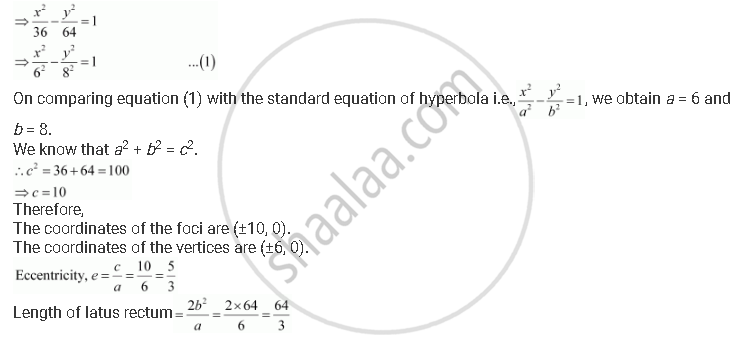CBSE (Arts) Class 11CBSE
Share
Notifications

View all notifications
Books Shortlist
Your shortlist is empty

# Find the Coordinates of the Foci and the Vertices, the Eccentricity, and the Length of the Latus Rectum of the Hyperbola 16x2 – 9y2 = 576 - CBSE (Arts) Class 11 - Mathematics

Login
Create free account

Forgot password?
ConceptHyperbola Eccentricity

#### Question

Find the coordinates of the foci and the vertices, the eccentricity, and the length of the latus rectum of the hyperbola 16x2 – 9y2 = 576

#### Solution

The given equation is 16x2 – 9y2 = 576.

It can be written as

16x2 – 9y2 = 576Is there an error in this question or solution?

#### APPEARS IN

Solution Find the Coordinates of the Foci and the Vertices, the Eccentricity, and the Length of the Latus Rectum of the Hyperbola 16x2 – 9y2 = 576 Concept: Hyperbola - Eccentricity.
S